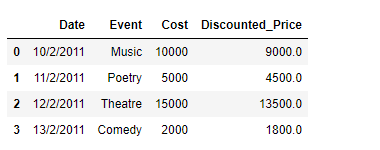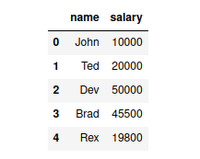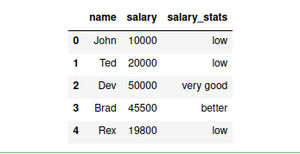GFG App
Open AppBrowser
Continue

# Create a new column in Pandas DataFrame based on the existing columns

While working with data in Pandas, we perform a vast array of operations on the data to get the data in the desired form. One of these operations could be that we want to create new columns in the DataFrame based on the result of some operations on the existing columns in the DataFrame. Let’s discuss several ways in which we can do that.

Given a Dataframe containing data about an event, we would like to create a new column called ‘Discounted_Price’, which is calculated after applying a discount of 10% on the Ticket price.

Example 1: We can use DataFrame.apply() function to achieve this task.

## Python3

 `# importing pandas as pd` `import` `pandas as pd`   `# Creating the DataFrame` `df ``=` `pd.DataFrame({``'Date'``:[``'10/2/2011'``, ``'11/2/2011'``, ``'12/2/2011'``, ``'13/2/2011'``],` `                    ``'Event'``:[``'Music'``, ``'Poetry'``, ``'Theatre'``, ``'Comedy'``],` `                    ``'Cost'``:[``10000``, ``5000``, ``15000``, ``2000``]})`   `# Print the dataframe` `print``(df)`

Output :Sample dataframe

Now we will create a new column called ‘Discounted_Price’ after applying a 10% discount on the existing ‘Cost’ column.

## Python3

 `# using apply function to create a new column` `df[``'Discounted_Price'``] ``=` `df.``apply``(``lambda` `row: row.Cost ``-` `                                  ``(row.Cost ``*` `0.1``), axis ``=` `1``)`   `# Print the DataFrame after addition` `# of new column` `print``(df)`

Output :Dataframe with new column created

Example 2: We can achieve the same result by directly performing the required operation on the desired column element-wise.

## Python3

 `import` `pandas as pd`   `# Creating the DataFrame` `df ``=` `pd.DataFrame({``'Date'``:[``'10/2/2011'``, ``'11/2/2011'``, ``'12/2/2011'``, ``'13/2/2011'``],` `                    ``'Event'``:[``'Music'``, ``'Poetry'``, ``'Theatre'``, ``'Comedy'``],` `                    ``'Cost'``:[``10000``, ``5000``, ``15000``, ``2000``]})`   `# Create a new column 'Discounted_Price' after applying` `# 10% discount on the existing 'Cost' column.`   `# create a new column` `df[``'Discounted_Price'``] ``=` `df[``'Cost'``] ``-` `(``0.1` `*` `df[``'Cost'``])`   `# Print the DataFrame after ` `# addition of new column` `print``(df)`

Output :Output DataFrame

Example 3: Using DataFrame.map() function to create new column from existing column using a mapping function

We will create a dataframe with some sample data:

## Python3

 `data ``=` `{` `    ``"name"``: [``"John"``, ``"Ted"``, ``"Dev"``, ``"Brad"``, ``"Rex"``, ``"Smith"``, ``"Samuel"``, ``"David"``],` `    ``"salary"``: [``10000``, ``20000``, ``50000``, ``45500``, ``19800``, ``95000``, ``5000``, ``50000``]` `}` `# create dataframe from data dictionary` `df ``=` `pd.DataFrame(data)` `# print the dataframe` `display(df.head())`

Output:Sample dataframe

Now, we will create a mapping function (salary_stats) and use the DataFrame.map() function to create a new column from an existing column

## Python3

 `def` `salary_stats(value):` `    ``if` `value < ``10000``:` `        ``return` `"very low"` `    ``if` `10000` `<``=` `value < ``25000``:` `        ``return` `"low"` `    ``elif` `25000` `<``=` `value < ``40000``:` `        ``return` `"average"` `    ``elif` `40000` `<``=` `value < ``50000``:` `        ``return` `"better"` `    ``elif` `value >``=` `50000``:` `        ``return` `"very good"`   `df[``'salary_stats'``] ``=` `df[``'salary'``].``map``(salary_stats)` `display(df.head())`

Output:Output dataframe with new column

Explanation: Here we have used pandas DataFrame.map() function to map each value to a string based on our defined mapping logic. The resultant series of values is assigned to a new column, “salary_stats”.

My Personal Notes arrow_drop_up